# `sklearn.cluster`.AgglomerativeClustering¶

class `sklearn.cluster.``AgglomerativeClustering`(n_clusters=2, affinity=’euclidean’, memory=None, connectivity=None, compute_full_tree=’auto’, linkage=’ward’, pooling_func=<function mean>)[source]

Agglomerative Clustering

Recursively merges the pair of clusters that minimally increases a given linkage distance.

Read more in the User Guide.

Parameters: n_clusters : int, default=2 The number of clusters to find. affinity : string or callable, default: “euclidean” Metric used to compute the linkage. Can be “euclidean”, “l1”, “l2”, “manhattan”, “cosine”, or ‘precomputed’. If linkage is “ward”, only “euclidean” is accepted. memory : None, str or object with the joblib.Memory interface, optional Used to cache the output of the computation of the tree. By default, no caching is done. If a string is given, it is the path to the caching directory. connectivity : array-like or callable, optional Connectivity matrix. Defines for each sample the neighboring samples following a given structure of the data. This can be a connectivity matrix itself or a callable that transforms the data into a connectivity matrix, such as derived from kneighbors_graph. Default is None, i.e, the hierarchical clustering algorithm is unstructured. compute_full_tree : bool or ‘auto’ (optional) Stop early the construction of the tree at n_clusters. This is useful to decrease computation time if the number of clusters is not small compared to the number of samples. This option is useful only when specifying a connectivity matrix. Note also that when varying the number of clusters and using caching, it may be advantageous to compute the full tree. linkage : {“ward”, “complete”, “average”}, optional, default: “ward” Which linkage criterion to use. The linkage criterion determines which distance to use between sets of observation. The algorithm will merge the pairs of cluster that minimize this criterion. ward minimizes the variance of the clusters being merged. average uses the average of the distances of each observation of the two sets. complete or maximum linkage uses the maximum distances between all observations of the two sets. pooling_func : callable, default=np.mean This combines the values of agglomerated features into a single value, and should accept an array of shape [M, N] and the keyword argument `axis=1`, and reduce it to an array of size [M]. labels_ : array [n_samples] cluster labels for each point n_leaves_ : int Number of leaves in the hierarchical tree. n_components_ : int The estimated number of connected components in the graph. children_ : array-like, shape (n_nodes-1, 2) The children of each non-leaf node. Values less than n_samples correspond to leaves of the tree which are the original samples. A node i greater than or equal to n_samples is a non-leaf node and has children children_[i - n_samples]. Alternatively at the i-th iteration, children[i] and children[i] are merged to form node n_samples + i

Methods

 `fit`(X[, y]) Fit the hierarchical clustering on the data `fit_predict`(X[, y]) Performs clustering on X and returns cluster labels. `get_params`([deep]) Get parameters for this estimator. `set_params`(**params) Set the parameters of this estimator.
`__init__`(n_clusters=2, affinity=’euclidean’, memory=None, connectivity=None, compute_full_tree=’auto’, linkage=’ward’, pooling_func=<function mean>)[source]
`fit`(X, y=None)[source]

Fit the hierarchical clustering on the data

Parameters: X : array-like, shape = [n_samples, n_features] The samples a.k.a. observations. y : Ignored self :
`fit_predict`(X, y=None)[source]

Performs clustering on X and returns cluster labels.

Parameters: X : ndarray, shape (n_samples, n_features) Input data. y : ndarray, shape (n_samples,) cluster labels
`get_params`(deep=True)[source]

Get parameters for this estimator.

Parameters: deep : boolean, optional If True, will return the parameters for this estimator and contained subobjects that are estimators. params : mapping of string to any Parameter names mapped to their values.
`set_params`(**params)[source]

Set the parameters of this estimator.

The method works on simple estimators as well as on nested objects (such as pipelines). The latter have parameters of the form `<component>__<parameter>` so that it’s possible to update each component of a nested object.

Returns: self :

## Examples using `sklearn.cluster.AgglomerativeClustering`¶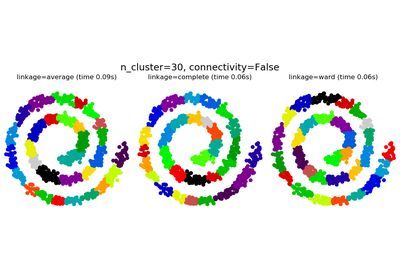Agglomerative clustering with and without structure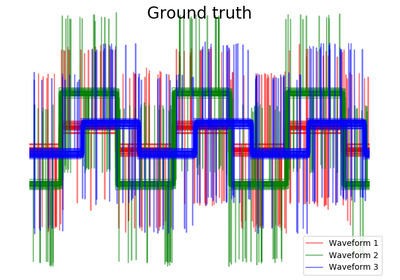Agglomerative clustering with different metrics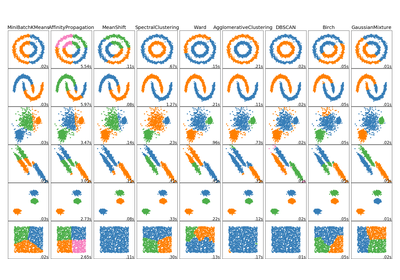Comparing different clustering algorithms on toy datasets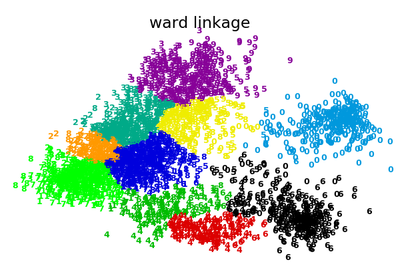Various Agglomerative Clustering on a 2D embedding of digits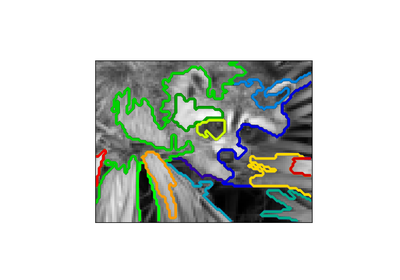A demo of structured Ward hierarchical clustering on a raccoon face image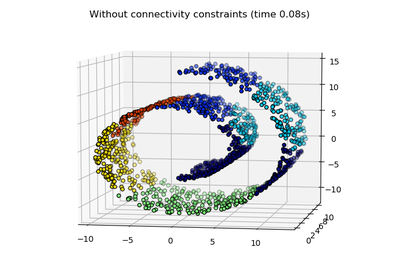Hierarchical clustering: structured vs unstructured ward